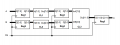# Calculating number of flip flops from a synchronus counter

#### Ajdawson94

Joined Nov 19, 2018
5this is an example from an exam to be used for my study the question is to calculate the number of flip flops... i know that this can be done using the in and out labeled and i know that the answer isf rom the I/O labels reg1= 8 bits, reg2 =4 bits, reg3= 8 bits, reg 4= 3 bits, reg 5 =12 bits so the Total flipflops = 8+4+8+3+12 = 35 however how do i take the i/o and get these corresponding bits?

for example for reg 1 do i take the 7 bit input and add the 0 for 8 bits? if so if i had lets say in reg x i had in (16:0) out(16:0) that would equate to 17 bits?

Last edited:

#### WBahn

Joined Mar 31, 2012
26,398
reg1 does not have a 7 bit input, it has an 8 bit input.

If I give you eight coins with the numbers 1 through 8 written on them (one number on each coin), how many coins have I given you? If I give you coins with the numbers 80 through 87, how many coins have I given you? If I give you coins numbered 0 though 7, how many have I given you?

If I have inputs numbered 0 through 7, how many inputs are there?